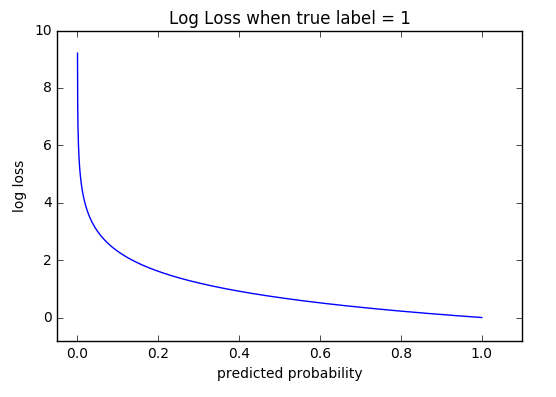# Loss Functions¶

## Cross-Entropy¶

Cross-entropy loss, or log loss, measures the performance of a classification model whose output is a probability value between 0 and 1. Cross-entropy loss increases as the predicted probability diverges from the actual label. So predicting a probability of .012 when the actual observation label is 1 would be bad and result in a high loss value. A perfect model would have a log loss of 0.The graph above shows the range of possible loss values given a true observation (isDog = 1). As the predicted probability approaches 1, log loss slowly decreases. As the predicted probability decreases, however, the log loss increases rapidly. Log loss penalizes both types of errors, but especially those predictions that are confident and wrong!

Cross-entropy and log loss are slightly different depending on context, but in machine learning when calculating error rates between 0 and 1 they resolve to the same thing.

Code

Math

In binary classification, where the number of classes $$M$$ equals 2, cross-entropy can be calculated as:

$-{(y\log(p) + (1 - y)\log(1 - p))}$

If $$M > 2$$ (i.e. multiclass classification), we calculate a separate loss for each class label per observation and sum the result.

$-\sum_{c=1}^My_{o,c}\log(p_{o,c})$

Note

• M - number of classes (dog, cat, fish)
• log - the natural log
• y - binary indicator (0 or 1) if class label $$c$$ is the correct classification for observation $$o$$
• p - predicted probability observation $$o$$ is of class $$c$$

## Hinge¶

Used for classification.

Code

## Huber¶

Typically used for regression. It’s less sensitive to outliers than the MSE as it treats error as square only inside an interval.

$\begin{split}L_{\delta}=\left\{\begin{matrix} \frac{1}{2}(y - \hat{y})^{2} & if \left | (y - \hat{y}) \right | < \delta\\ \delta ((y - \hat{y}) - \frac1 2 \delta) & otherwise \end{matrix}\right.\end{split}$

Code

Further information can be found at Huber Loss in Wikipedia.

Code

## RMSE¶

Root Mean Square Error

$RMSE = \sqrt{\frac{1}{m}\sum^{m}_{i=1}(h(x^{(i)})-y^{(i)})^2}$
RMSE - root mean square error
m - number of samples
$$x^{(i)}$$ - i-th sample from dataset
$$h(x^{(i)})$$ - prediction for i-th sample (thesis)
$$y^{(i)}$$ - ground truth label for i-th sample

Code

## MAE (L1)¶

Mean Absolute Error, or L1 loss. Excellent overview below  and .

$MAE = \frac{1}{m}\sum^{m}_{i=1}|h(x^{(i)})-y^{(i)}|$
MAE - mean absolute error
m - number of samples
$$x^{(i)}$$ - i-th sample from dataset
$$h(x^{(i)})$$ - prediction for i-th sample (thesis)
$$y^{(i)}$$ - ground truth label for i-th sample

Code

## MSE (L2)¶

Mean Squared Error, or L2 loss. Excellent overview below  and .

$MSE = \frac{1}{m}\sum^{m}_{i=1}(y^{(i)} - \hat{y}^{(i)})^2$
MSE - mean square error
m - number of samples
$$y^{(i)}$$ - ground truth label for i-th sample
$$\hat{y}^{(i)}$$ - predicted label for i-th sample

References